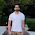## Thursday, 16 February 2017

### Hidden Markov Model- Session 1

Hidden Markov Models are used in temporal( time-series) pattern recognition. They are used in speech, handwriting, gesture and text recognition. Generally known as HMM, they follow Markovian assumption. According to Markov assumption( Markov property) , future state of system is only dependent on present state.

In HMM, time series' known observations are known as visible states. And It is assumed that these visible values are coming from some hidden states. The machine learning algorithms today identify these things in a hidden markov model-

1) Hidden state sequence
2) Transition probabilities
3) Emission value( visible state) distribution.

## Let us run this algorithm for physician's behaviour-

physician_prescrition_data <-c(12,16,45,45,56,67,78,98,120,124,156)

physician_prescrition_data , contains time series of its prescription values which are 12, 16, 45....

> mod <- depmixS4::depmix(physician_prescrition_data~1, nstates = 2, ntimes = 11)
> fm <- fit(mod)

model execution would give many parameters, lets focus on only 3 mentioned above.

1) Transition probabilities are probabilities of movement between hidden states. As we have given 2 state model in mod variable, we will have 2 hidden states; say s1 and s2. So there will be four transition probabilities-
s1 to s1 transition- 1.000000e+00
s1 to s2 transition- 5.039408e-52
s2 to s1 transition- 0.1657764
s2 to s2 transition-  0.8342236

2) Hidden State Sequence- What was the sequence of hidden states that have produced the given o/p values/ Visible values. This is calculated using Viterbi Algo.
For our prescription data the hidden sequence is-

S1 ans S2 are the participation probabilities of S1 ans S2 at every time period. If you see at sequence no 7, s1 is present with .22 and s2 is present with .77 probability. Maximum of s1 and s2 is selected as hidden state for that time period.

3) Emission Values- Emission Value distribution and transition probabilities are calculated by Forward-backward Algo / Baum Welch Algo. Emission values are the distribution of visible state from any hidden state. We have 2 hidden states so there will be 2 output distribution.

We have got 2 normal distribution with mean values of 114 and 40. So from S1 output would be 114 +-27 and from S2 it would be 20+- 20.

This is how different Hidden Markov models work.Here we can identify hidden states associated with physician. He is high prescribing at S1 and low at s2, I had taken continuously increasing data so initially all are s2 and remaining are S1. Other data values would give more meaningful information. Please let me know if you want more clarification on any point I had mentioned in blog.

further reading to understand evaluation problem of HMM-

R Code snippets-

## Required library
library(depmixS4)

physician_prescrition_data <-c(12,16,45,45,56,67,78,98,120,124,156)

## model execution-
HMM_model <- depmixS4::depmix(physician_prescrition_data~1, nstates = 2,ntimes=length(physician_prescrition_data))

## model fitting
HMM_fm <- fit(HMM_model)

## Transition probabilties-
HMM_fm@transition

## posterior states-
posterior(HMM_fm)
plot(ts(posterior(HMM_fm)[,1]))

## Emission probabilties-
HMM_fm@response

Same is available at Github-

1.HMM
simple and nice explanation.
on prices observations:
how to "translate" 2( or 3) hidden states , to let say:
predict next price on time t+1 with height's probability ?

2.I haven't understood completely. but if you are talking about next observation value, u can follow this-
http://machinelearningstories.blogspot.com/2017_03_01_archive.html

Number of hidden state is calculated by least AIC criteria.

3.Do you know how to convert Excel data into Time series data. I have maternity data and want to run HMM on it? and Is it necessary to convert your data in time series to run HMM on it?

1.yes, data should be in sequence to apply HMM.Load excel data into R/Python and run HMM.

4.Hello, im doing a project on debit card fraud detection, The data i have are bank transactions so i need help on how to apply these transations to the HMM. And also how i can simulate more transations for the sack of trainning the HMM.. Please Help.

5.U can do monte Carlo simation to generate more such data.
U can apply hmm on your data but I am not sure how hmm will help in fraud detection